# Decision Tree Learning

Types of machine learning:

Machine learning algorithms can be organized into a taxonomy based on the desired outcome of the algorithm. 

1. Supervised learning: Generates a function that maps inputs to desired outputs (also called labels). This is most common type of learning.
2. Unsupervised learning: Algorithm tries to identify similarities between the inputs so that inputs that  have something in common are categorized together.
3. Reinforcement learning: Learns how to act given an observation of the world. Every action has some impact in the environment and the environment provides feedback in the form of rewards/penalizes that guides the learning algorithm. The algorithm gets told when the action is wrong but does not get told how to improve it.

Supervised Learning:

For a given set of inputs and outputs, the algorithms in supervised learning tries to find a function that  maps inputs to desired outputs. Once the algorithm is modeled we could use it to address either of the below two types of problems.

a) Regression Problem:

For a given input in data set, the algorithm fits a mathematical function describing a curve, so that curve passes as close as possible to all of the data points. When a new data point is given outside of earlier training input, then we could anticipated output by interpolating on the curve that fits out model.

Examples:

• Real Estate: For a given set of training data containing # of bed rooms, total square foot, # of bath rooms, city / state; we could have current market value of the house as output / target value. By training the system on the above data, we could solve regression problem of estimating how much a house of 1 Bed, 1 Bath in local vicinity could be costing in current market.

b) Classification Problem:

The classification problem consists of learning how to classify the input as which of the N classes it belong to based on the training it got from example / sample data. The most important point about the classification problem is that it is discrete, each example belongs to precisely one class. Once the system has learnt the model from sample data, it would be possible to classify a new data accordingly.

Examples:

• Health Care: For a given set of health metrics such as blood pressure, heart rate, glucose / sugar and cholesterol levels, etc. classify the patient’s risk (high, medium, low or none) of having heart disease in future.

Most interesting point to note here would be, if an algorithm is know to solve one of the problem, then it could be tweaked to solve the other problem as well.

Decision Tree Learning

Decision tree learning uses a decision tree as a predictive model which maps observations about an item to conclusions about the item’s target value . Decision tree learning algorithms could be used to solve either classification and/or regression problems.

In data mining for a given set of sample data, containing features & labels, the decision tree algorithms help create a model to predict what would be the outcome / label of test data. In the below sample data, we are looking for “What should be the activity in the evening” based on attributes such having a project deadline, feeling lazy and if there is a party to night.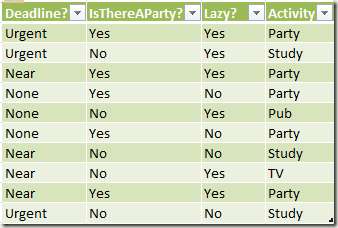Once the above data is fed into the ID3 decision tree algorithm, it generates a model as show below, where the leaf nodes are activities and all other nodes drive the conditional logic based on the other attribute values.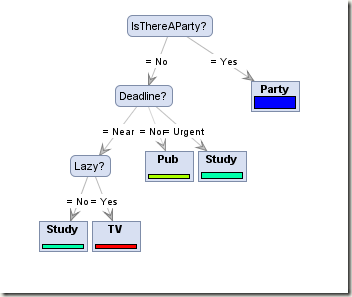The advantages of Decision Tree algorithms are

• They are easy to understand and interpret
• Requires little sample data for preparing the model
• Able to handle both numerical and categorical data values
• Lower cost, O log(N), to query/run through the model

At the same time, the disadvantages of Decision Tree algorithms is that

• Tend to create over complex trees for large / complex data sets (also called over fitting)
• Algorithm is prone to generating locally optimal decision tree (within the given sample) but does not guarantee globally optimal decision tree (complete data set from sample, validation and test data set)

Constructing Decision Trees:

There are a few different decision tree algorithms, but they are almost all variants of same principle: the algorithms build the tree in a greedy manner starting at the root, choosing the most informative feature at each step. Most commonly used algorithms are ID3, C4.5 and CART.

Assuming that we could encode everything as 0 (Negative) and 1 (Positive); The algorithms learns to classify the data based on various attributes (DeadLine?, IsThereAParty?, Lazy?) and their values. If an attribute is always positive, then not much information is gained over it as it tends to be always positive. However, if an attribute (and/or with conditional logic) is found in such a way we have 50% positive and 50% negative then the system has gained “maximum information entropy” by using that attribute. Example of such attribute is our root node with “IsThereAParty?”

A function for computing the entropy is

ƒ(x) : (x == 0) ? 0 : – x * log2(x); where x is probability of value in all possible values of a attribute

ID3 Algorithm

The idea of ID3 algorithm is to work out how much the entropy of the whole training set would decrease if we choose each particular attribute for the next classification step. This is know as the information gain, and is defined as entropy of the whole example set (S) minus the entropy when a particular feature (f) is chosen.

Gain(S, F) = Entropy (S) – (|Sf|/|S|) * Entropy(Sf); where f values of feature F

|Sf| – represents # of members in sample data S that have value f for feature F.Using the above data .. Entropy (Sη) for whole sample set is

Entropy(Sη) = Entropy(Party) + Entropy(Study) + Entropy(Pub) + Entropy(TV)

Out of the 10 sample data set, we have 5 Party outcomes. Hence is pParty 5/10. Therefore Entropy(Party) is

Entropy(Party) = – pParty * log2(pParty) = –0.5 * log2(0.5) = 0.5
Entropy(Study) = – pStudy * log2(pStudy) = –0.3 * log2(0.3) = 0.5211
Entropy(Pub) = – pPub * log2(pPub) = –0.1 * log2(0.1) = 0.3322
Entropy(TV) = – pTV * log2(pTV) = –0.1 * log2(0.1) = 0.3322

resulting in Entropy(Sη) = 1.6855 … 

then we find which feature has the maximum information gain.

The information gain we could have with DeadLine as the root node of the decision tree is:

Gain(S, DeadLine) = Entropy(Sη) – (|Sf|/|S|) Entropy(Sf);
where f values (Urgent, Near, None) of feature DeadLine

Out of the given 10 records, we got 3 records with DeadLine value as Urgent (also shown below). Out of these three, we got Activity recommendations as Study (2 instances) and Party (1 instance).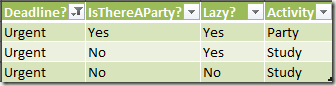Entropy(Sη)
– |SD.Urgent|/|Sη| * Entropy(SD.Urgent)
– |SD.Near|/|Sη| * Entropy(SD.Near)
– |SD.None|/|Sη| * Entropy(SD.None)

However, Entropy(SD.Urgent) is turn Entropy(SDU.Party) + Entropy(SDU.Study)
where DU.Party probability of Activity being Party when DeadLine is Urgent and |Sη| is total number of rows in the given data set.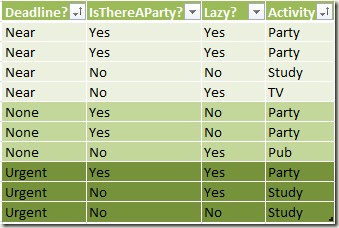Entropy(SDU.Party) = – pDU.Party * log2(pDU.Party) = -1/3 * log2(1/3)
Entropy(SDU.Study) = – pDU.Study* log2(pDU.Study) = -2/3 * log2(2/3)
Entropy(SD.Urgent) = -1/3 * log2(1/3) + -2/3 * log2(2/3) = -0.91829 … 

Similarly …

Entropy(SD.Near) =  Entropy(SDN.Party) + Entropy(SDN.Study) + Entropy(SDN.TV)
Entropy(SDN.Party) = – pDN.Party * log2(pDN.Party) = 2/4 log2(2/4)
Entropy(SDN.Study) = – pDN.Study* log2(pDN.Study) = 1/4 log2(1/4)
Entropy(SDN.TV) = – pDN.TV* log2(pDN.TV) = 1/4 log2(1/4)
Entropy(SD.Near) =  -2/4 * log2(2/4) + -1/4 * log2(1/4) + -1/4 * log2(1/4) = -1.5 … 

Entropy(SD.None) =  2/3 * log2(2/3) + 1/3 * log2(1/3) = -0.91829 … 

Now using all of data values from  through 

Gain(S, DeadLine) = 1.6855 – (3/10 * -0.9183 + 4/10 * -1.5 + 3/10 * -0.9183)
Gain(S, DeadLine) = 0.5345 … 

Computing the same for information Gain by using Party as the root node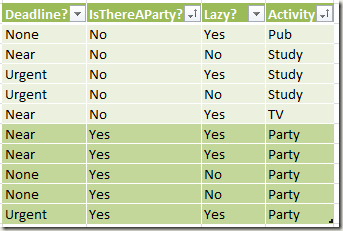Gain(S, Party) =

Entropy(Sη
– |SP.Yes|/|Sη| * Entropy(SP.Yes
– |SP.No|/|S?| * Entropy(SP.No)

recollect that
Entropy(SP.No) = Entropy(SPN.Pub) + Entropy(SPN.Study) + Entropy(SPN.TV) and
Entropy(SP.Yes) = Entropy(SPY.Party)

= 1.6855  – 5/10 * (-5/5 * log2(5/5)) – 5/10 * (-1/5 * log2(1/5) -3/5 * log2(3/5)  -1/5 * log2(1/5))

Gain(S, Party) = 1.0 … 

Computing the same for information Gain by using Party as the root node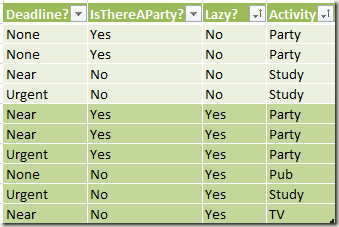Gain(S, Lazy) = 1.6855 – 4/10 * (-2/4 * log2(2/4) – 2/4 * log2(2/4)) – 6/10 * (-3/6 * log2(3/6) – 1/6 * log2(1/6) – 1/6 * log2(1/6) – 1/6 * log2(1/6)) = 0.21 …

So based on ,  and  maximum information is gained by using Party as the root node. There are only two values for the Party (Yes and No) hence there would be two branches coming out of it. When you look at Party.Yes node, we have Activity as Party for all 5 of them. Therefore we just put a leaf node recommending .. ‘goto Party’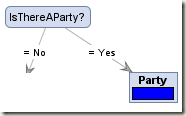Now comes the interesting party. What should be done when there is ‘No Party’.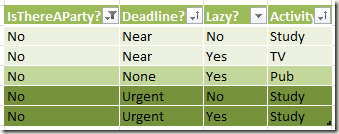We compute how much of information is gained by using DeadLine or Lazy as child node for when Party is No.

SPN.DeadLine = Entropy(SPN) – |SPN.DNear| / |SPN| * Entropy(SPN.DNear) – SPN.DNone/ |SPN| * Entropy( SPN.DNone) – |SPN.DUrgent| / |SPN| * Entropy( SPN.DUrgent)

where …

Entropy(SPN) is Entropy when Party is No
|SPN| total number records with Party as No.
SPN.DUrgent represents data with Deadline value as Urgent when Party is No and

SPN = Entropy(SPN.Study) + Entropy(SPN.TV) + Entropy(SPN.Pub)
= -3/5*log2(3/5) -1/5*log2(1/5) -1/5*log2(1/5) = 1.371 …

also …
SPN.DNear = Entropy(SPN.DN.Study) + Entropy(SPN.DN.TV) + Entropy(SPN.DN.Pub)

SPN.DeadLine =  1.371 – 2/5 * (-1/2*log2(1/2) -1/2*log2(1/2)) – 1/5 * (-1/1*log2(1/1)) – 2/5 * (-2/2*log2(2/2)) = 0.971 … 

SPN.Lazy = 1.371 – 3/5(-1/3*log2*(1/3) -1/3*log2*(1/3) -1/3*log2*(1/3)) – 2/5(-2/2*log2*(2/2)) = 0.583 … 

Comparing  and  we gain more information with DeadLine as child node of when ‘Party is No’. Since DeadLine has three Values (Near, None, Urgent) we branch out three nodes and with ‘None’ and ‘Urgent’ containing all same values (no new information could be gained) we could have ‘Study’ as leaf node under ‘Urgent’ and ‘Pub’ as leaf node for ‘None’ resulting in tree as shown here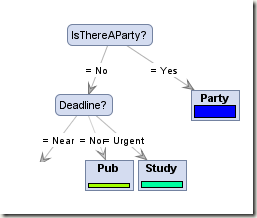Similarly, when ‘No Party’ and ‘Near DeadLine’ we have two rows with two Activities (Study & TV) as outputs. Since each of them are unqiue based on whether one is Lazy or not, we could just a another Lazy node as the decision factor leading to other leaf nodes as in hereReferences:

## One thought on “Decision Tree Learning”

1.rakesh on said:

I came across your blog while doing a research on decision tree, how would you handle if there is missing info.

For example you don’t have info for the deadline for some of the rows.
<>
<>
<>
<>

(? indicates a missing value)